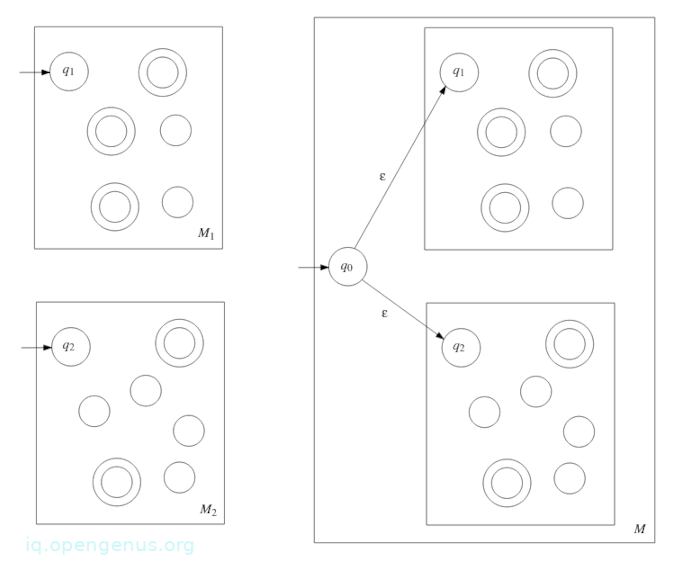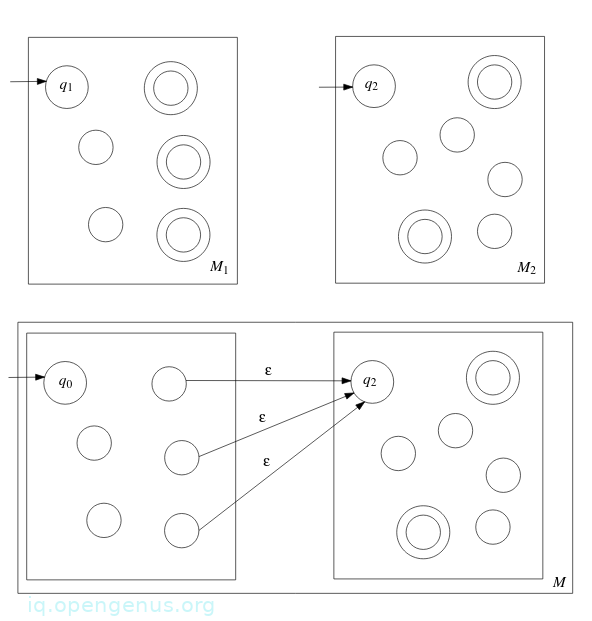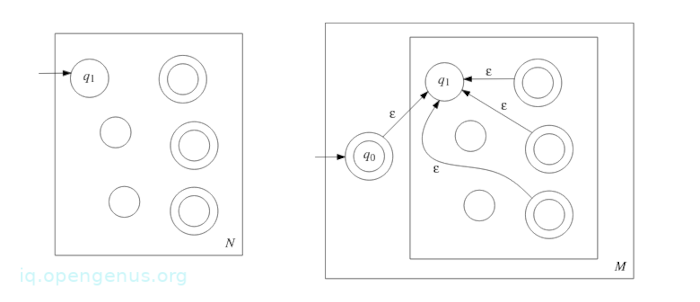×

Search anything:

# Regular Operations in Theory of Computation

#### Theory of ComputationIn this article we have discussed three languages operations namely, union, concatenation and kleen closure.

1. Introduction.
2. Regular operations.
3. Concatenation and Kleen closure operations.
4. Closure under regular operations
5. Summary.
6. References.

## Introduction.

A language is a set of strings from an a finite or infinite alphabet. Here we discuss three simple but important operations used on languages, these are union, concatenation and kleen closure.

Others include, intersection and difference.

## Regular operations.

There are three operations which are performed on languages namely: union, concatenation and kleen closure.

Let A and B be two languages over a similar alphabet:

1. The union of A and B is defined as:
A ∪ B = {w : w ∈ A or w ∈ B}

2. The concatenation of the two is defined as:
AB = {ww′ : w ∈ A and w′ ∈ B} where AB is the set of all strings obtained by taking an arbitrary string w in A and an arbitrary string w′ in B then putting them together such that the former is to the left of the latter.

3. The kleen closure of A is defined as:
A* = {${\mathrm{u}}_{\mathrm{1}}$${\mathrm{u}}_{\mathrm{2}}$ ... ${\mathrm{u}}_{\mathrm{k}}$ : k ≥ 0 and ${\mathrm{u}}_{\mathrm{i}}$ ∈ A for all i = 1, 2, ..., k}
Where A* is obtained by taking an infinite number of strings in A and putting them together.
Note that k cannot be zero, in this case it will correspond to an empty string ϵ and therefore ϵ ∈ A*.

An Example:
Let A = {0, 01} and B = {1, 10}.
union: A ∪ B= {0, 01, 1, 10}
concatenation: AB = {01, 010, 011, 0110}
kleen closure: A* = {ϵ, 0, 01, 00, 001, 010, 0101, 000, 0001, 00101, ...}

An example:
If Σ = {0, 1}, Σ* is the set of all binary strings including an empty string.

Alternative kleen closure definition for a language A,
${\mathrm{A}}^{0}$ = {ϵ}.
For k ≥ 1, ${\mathrm{A}}^{k}$ = ${\mathrm{AA}}^{k-1}$ where ${\mathrm{A}}^{k}$ is a concatenation of the languages A and ${\mathrm{A}}^{k-1}$.
We define A* as follows:
A* = $\bigcup _{\mathrm{k}=0}^{\infty }{\mathrm{A}}^{\mathrm{k}}$

Theorem 1: The set of regular languages is closed under the union operation, that is, if A and B are regular languages over a similar alphabet Σ, A ∪ B is a regular language.

Proof: A and B are regular languages, therefore there are finite automata M1 = (Q1, Σ, δ1, q1, F1) and M2 = (Q2, Σ, δ2, q2, F2) that accept A and B respectively.
The next step is to construct a finite automaton M that accepts A ∪ B so as to prove A ∪ B is regular.
M must have the following property:
for every string w ∈ Σ*, M accepts w ⇔ M1 accepts w or M2 accepts w.

Idea:
Assuming M could do the following:

• Starting in the start state q1 of M1, M runs M1 on w.
• After reading w, M1 is in the F1 state, then w ∈ A and therfore w ∈ A ∪ B and so M accepts w.
• If after reading w, M1 is in the state other than F1, w ∉ A and M runs M2 on w, starting in the start state q2 of M2. If after reading w, M2 is in F2 state, we know w ∈ A ∪ B and so M accepts w. Otherwise w ∉ A ∪ B and M rejects w.

This idea won't work since the finite automaton M reads the input string once.

A better approach is to run M1 and M2 simultaneously.
First, we define the set Q of states of M as the cartesian product Q1 x Q2. If M is in the state (r1, r2), this indicates that;

• If M1 reads the input string to this point, it will be in r1 state.
• If M2 reads the input string to the same point, it will be in r2 state.

An therefore we have the finite automaton M = (Q,Σ, δ, q, F) where:

• Q = Q1 x Q2 = {(r1, r2) : r1 ∈ Q1 and r2 ∈ Q2}, note that |Q| = |Q1| x |Q2| and this is finite.
• Σ is the alphabet of A and B, here we assume the both A and B are languages over the same alphabet.
• M's start state q is equal to (q1, q2), i.e, q = (q1, q2).
• Set F of accept states of M is: F = {(r1, r2) : r1 ∈ F1 or r2 ∈ F2} = (F1 x Q2) ∪ (Q1 x F2).
• The transition function δ : Q x Σ → Q is:
δ((r1, r2), a) = (δ1(r1, a), δ2(r2, a)), for all r1 ∈ Q1, r2 ∈ Q2, a ∈ Σ

We complete the proof by showing that this finite automaton M accepts the language A ∪ B. This is clear from what we seen from above.

To give a formal proof we use extended transition functions $\stackrel{-}{\delta 1}$ and $\stackrel{-}{\delta 2}$.
Now we have to prove:
M accepts w ⇔ M1 accepts w or M2 accepts w.
That is, M accepts w ⇔ $\stackrel{-}{\delta 1}$(q1, w) ∈ F1 or $\stackrel{-}{\delta 2}$(q2, w) ∈ F2.

And in terms of the extended transition function $\stackrel{-}{\delta }$ of the transition function δ of M, it becomes:

$\stackrel{-}{\delta }$((q1, q2),w) ∈ F ⇔ $\stackrel{-}{\delta 1}$(q1, w) ∈ F1 or $\stackrel{-}{\delta 2}$(q2, w) ∈ F2

By applying the extended transition function definition we can see that
$\stackrel{-}{\delta }$((q1, q2), w) = ($\stackrel{-}{\delta 1}$(q1, w), $\stackrel{-}{\delta 2}$(q2, w)).
The above implies that the former is true and thus M accepts the language A ∪ B.

## Concatenation and Kleen closure operations.

Theorem 2: Regular languages are closed under concatenation and kleen closure operations and we shall see in the proof below:

Proof: Let A and B be two languages and M1 and M2 be finite automata that accept both languages respectively.

Now to construct a finite automaton M that accepts the concatenation of AB.
Let u be an input string, M has to decide whether it can be broken into two strings w and w′ such that
w ∈ A and w′ ∈ B.
In other words, M has to decide whether or not u can be broken down into two substrings such that the first is accepted by M1 and the second is accepted by M2.

The complexity comes in where by M has to make the decision by scanning the string only once.
If u ∈ AB, then M decides if it can be broken and where to break u into two substrings such that the first substring is in the A and the second is in B, similarly if u ∉ AB, M has to decide that the string u cannot be broken into substrings all this is done in a single scan of the input string.

It is even more difficult to prove A* is a regular language if A is regular since for this proof we need a finite automaton that when given an arbitrary input string u, decides whether it can be broken into substrings such that each substring is in A.
The issue is that the finite automaton u ∈ A* has to determine the number of substrings and where to break and do this in a single scan of u.

As previously stated, if A and B are regular languages, both AB and A* are also regular.
To prove this claim we use a more general type of finite automaton, Non-deterministic finite automaton which we have discussed in the prerequisite article.
With this automaton we can easily prove the theorem that the class of regular languages is closed under the concatenation and kleen closure operations.

## Closure under regular operations.

We have the following theorem from the prerequisite article.
Theorem 3: Let A be a language. Then A is regular if and only if there exists a nondeterministic finite automaton that accepts A.

Here we will use the concept of NFA from the prerequisite article together with the above theorem to to prove that regular languages are after all closed under regular operations.

An alternative proof of theorem 1:
Theorem 1.1: The set of regular languages is closed under the union operation, that is, if A1 and A2 are regular languages over a similar alphabet Σ, then A1 ∪ A2 is also a regular language.

Proof: Since A1 is regular, by theorem 3: and NFA M1 = (Q1,Σ, δ1, q1, F1) such that A1 = (LM1).
Similarly, there exists a NFA M2 = (Q2,Σ, δ2, q2, F2) such that A2 = L(M2).
We also assume that Q1 ∩ Q2 = ∅ because otherwise we can give new names to states Q1 and Q2.
From the two NFAs we construct a NFA M = (Q,Σ, δ, q0, F) such that L(M) = A1 ∪ A2NFA M is defined as:

1. Q ={q0} ∪ Q1 ∪ Q2 where q0 is a new state.
2. q0 is the start state of M.
3. F = F1 ∪ F2
4. δ : Q × Σϵ → P(Q) where for any r ∈ Q and a ∈ Σϵ

*δ(r, a) = {

Theorem 4:
The set of regular languages is closed under the concatenation operation, that is, if A1 and A2 are regular languages over a similar alphabet Σ, then A1A2 is also a regular language.

Proof: Let M1 = (Q1,Σ, δ1, q1, F1) be an NFA such that A1 = L(M1). Similarly, let M2 = (Q2,Σ, δ2, q2, F2) be an NFA such that *A2 = L(M2. From above proof of theorem 1.1 we assume that Q1 ∩ Q2 = ∅ and therefore construct an NFA M = (Q,Σ, δ, q0, F) such that L(M) = A1A2.NFA M is defined as follows,

1. Q = Q1 ∪ Q2.
2. q0 = q1
3. F = F2
4. δ : Q × Σϵ → P(Q), where for any r ∈ Q and a ∈ Σϵ

δ(r, a) = {

Theorem 5: The set of regular languages is closed under the kleen closure operation, that is, If A is a regular language, the A* is also a regular language.

Proof: Let Σ be the alphabet of A and N = (Q1,Σ, δ1, q1, F1) be a NFA such that A = L(N). We now construct a NFA M = (Q,Σ, δ, q0, F) such that L(M) = A*.We define it as follows,

1. Q = {q0} ∪ Q1 where q0 is a new state.
2. q0 is the start state of M.
3. F = {q0} ∪ F1, (considering that ϵ ∈ A* has to be an accept state)
4. δ : Q × Σϵ → P(Q), where for any r ∈ Q and a Σ&#x3f5

δ(r, a) = {

Theorem 6: The set of regular languages is closed under the complement and intersection operations:

1. If a language A is over an alphabet Σ, the complement $\stackrel{-}{\mathrm{A}}$ = {w ∈ Σ* : w ∉ A} is also a regular language.
2. If A1 and A2 are regular languages over a similar alphabet Σ the intersection A1 ∩ A2 = {w ∈ Σ*: w ∈ A1 and w ∈ A2} is also a regular language.

## Summary.

We have discussed the union of two languages for example A and B which produce a set of strings either in A or B or both is written as:

A ∪ B = {w : w ∈ A or w ∈ B}

Their concatenation is denoted by AB = {ww′ : w ∈ A and w′ ∈ B} where AB is the set of all strings obtained by taking an arbitrary string w in A and an arbitrary string w′ in B then putting them together such that the former is to the left of the latter.

A kleen closure refers to zero or more occurrences of symbols in a string, it also includes an empty string ϵ which has a length of zero.

#### Erick Lumunge

Erick is a passionate programmer with a computer science background who loves to learn about and use code to impact lives positively.

Regular Operations in Theory of Computation